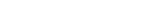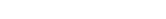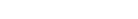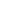How Cheenta works to ensure student success?
Explore the Back-Story

# TOMATO Objective 21 | ISI Entrance ExamThis is a problem from TOMATO Objective 21 based on positive integers. This problem is helpful for ISI Entrance Exam. Try out the problem.

Problem: TOMATO Objective 21

Suppose x & y are positive integers, x>y, and 3x+4y & 2x+3y when divided by 5, leave remainders 2&3 respectively. It follows that when (x-y) is divided by 5, the remainder necessarily equals
(a) 2 (b)1 (c) 4 (d) none of these

According to the problem,subtracting the above 2 relations we get,i.e.Hence the remainder is.

ISI Entrance Course

ISI Entrance Problems and Solutions

Inequality with Twist – Video

This is a problem from TOMATO Objective 21 based on positive integers. This problem is helpful for ISI Entrance Exam. Try out the problem.

Problem: TOMATO Objective 21

Suppose x & y are positive integers, x>y, and 3x+4y & 2x+3y when divided by 5, leave remainders 2&3 respectively. It follows that when (x-y) is divided by 5, the remainder necessarily equals
(a) 2 (b)1 (c) 4 (d) none of these

According to the problem,subtracting the above 2 relations we get,i.e.Hence the remainder is.

ISI Entrance Course

ISI Entrance Problems and Solutions

Inequality with Twist – Video

This site uses Akismet to reduce spam. Learn how your comment data is processed.

### 3 comments on “TOMATO Objective 21 | ISI Entrance Exam”

1.alisha says:

The question says 3x+4y and you have solved the problem with 3x+2y (as on 6 may 2015). According to the question shouldn't the answer be 1?

1.dawnlilacs says:

I checked the question in my book. As per to book, in question there should be 3x+2y instead of 3x+4y. So answer is 4 and in question there is typing mistake!

2.Matrika Shukla says:

3x + 2y = 5p + 2, where p is an integer. ---------- (1)
[Since given 3x + 2y leaves remainder 2 when divided by 5]

Similarly, 2x + 3y = 5q + 3 -------- (2)

(1) - (2): x - y = 5(p - q) - 1
==> The remainder is -1, = -1 + 5 = 4

Thus when (x - y) is divided by 5, remainder is 4.
I think this is the solution !

### Knowledge Partner